## New Maths Curriculum: Year 1 Multiplication and Division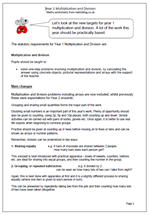Let’s look at the new targets for year 1 multiplication and division. A lot of the work this year should be practically based.

The statutory requirements for Year 1 Multiplication and Division are:

Multiplication and division

Pupils should be taught to:

•    solve one-step problems involving multiplication and division, by calculating the answer using concrete objects, pictorial representations and arrays with the support of the teacher.

Main changes

Multiplication and division problems including arrays are now included, whilst previously these were expectations for Year 2 onwards.

Grouping and sharing small quantities forms the major part of the work.

Doubling small numbers is an important part of this year’s work. Plenty of opportunity should also be given to counting, using 2p, 5p and 10p pieces, both counting up and down. Similar activities can be carried out with pairs of socks, gloves etc. Once again, it is better to use real life objects when beginning to combine groups.

Practice should be given at counting up in twos before moving on to fives or tens and can be shown as arrays or number patterns.
Remember, division can be understood in two ways:

1. Sharing equally               e.g. 6 bars of chocolate are shared between 2 people.
How many bars does each person get?

This concept is best introduced with practical apparatus – bowls of sweets, counters, buttons etc. are ideal for sharing into equal groups, and then counting the number in the group.

2. Grouping, or repeated subtraction                     e.g. 8 divided by 2
can be seen as how many lots of two can I take from eight?

Again, this is best done with apparatus at first and it is a slightly different process to sharing equally (where one item is given to each person in turn).

This can be answered by repeatedly taking two from the pile and then counting how many lots of two have been taken altogether.

Multiplication and division in Year 1

## New Maths Curriculum: Year 1 Addition and Subtraction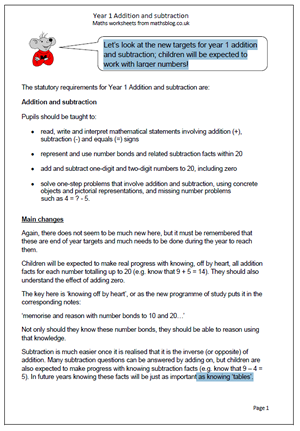Let’s look at the new targets for year 1 addition and subtraction; children will be expected to work with larger numbers!

Pupils should be taught to:

•    read, write and interpret mathematical statements involving addition (+), subtraction (-) and equals (=) signs

•    represent and use number bonds and related subtraction facts within 20

•    add and subtract one-digit and two-digit numbers to 20, including zero

•    solve one-step problems that involve addition and subtraction, using concrete objects and pictorial representations, and missing number problems
such as 4 = ? – 5.

Again, there does not seem to be much new here, but it must be remembered that these are end of year targets and much needs to be done during the year to reach them.
Children will be expected to make real progress with knowing, off by heart, all addition facts for each number totalling up to 20 (e.g. know that 9 + 5 = 14). They should also understand the effect of adding zero.
The key here is ‘knowing off by heart’, or as the new programme of study puts it in the corresponding notes:
‘memorise and reason with number bonds to 10 and 20…’
Not only should they know these number bonds, they should be able to reason using that knowledge.
Subtraction is much easier once it is realised that it is the inverse (or opposite) of addition. Many subtraction questions can be answered by adding on, but children are also expected to make progress with knowing subtraction facts (e.g. know that 9 – 4 = 5). In future years knowing these facts will be just as important as knowing ‘tables’.

New programme of Study: Year 1 Addition and Subtractionddition and subtraction

## New Maths Curriculum: Year 1 Number and Place ValueIt’s a whole new set of targets for Year 1 from 2014. Let’s begin by having a look at Number and Place Value.

The statutory requirements for Year 1 Number and Place Value are:

Number and place value

Pupils should be taught to:

•    count to and across 100, forwards and backwards, beginning with 0 or 1, or from any given number

•    count, read and write numbers to 100 in numerals; count in multiples of twos, fives and tens

•    given a number, identify one more and one less

•    identify and represent numbers using objects and pictorial representations including the number line, and use the language of: equal to, more than, less than (fewer), most, least

•    read and write numbers from 1 to 20 in numerals and words.

Main changes

The new maths programme of study is much shorter than previous models with much broader statements. This makes it tricky to know what has been left out. However, the main aim is to raise standards by raising targets to be achieved. In Year 1 the main changes are:

1. Counting, reading and writing numbers to 100 rather than just up to 20. This will prove to be very difficult for many children and will need a great deal of practice.
2. Perhaps even harder is to count in twos, fives and tens from different multiples.

New Statutory Requirements 2014:  Number and Place Value

## Perpendicular lines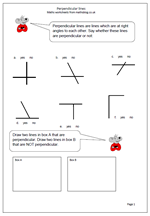The term perpendicular was usually introduced to children in the upper primary years and indeed into secondary school, but it is now thought that it should be introduced in year 3. At this stage children just need to be able to recognise two lines which are perpendicular to each other and to make it easier all the pairs of lines shown on the worksheet either cross or touch.

Of course, the key to being able to do this is to recognise a right angle and plenty of practice with recognising right angles must be done before going on to using the term perpendicular.   When the lines are vertical and horizontal it is quite easy to spot perpendicular lines but other placements make it more difficult to see just by looking and there is no harm in using the corner of a piece of paper to judge whether this is the case.

Perpendicular lines

## Year 6 resource of the week: factors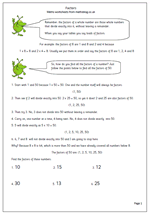Understanding factors and multiples is something many children fail to grasp. Here we have a page on factors and how to find all the factors of a number. The process is fairly straightforward, but can be quite time consuming. A good knowledge of tables and division is also needed. So if children’s knowledge of tables is weak, if they find division difficult and if they have little staying power then it is unlikely that they will enjoy trying to find factors of numbers!

One point that even brighter children take a while to understand is that you only need to continue to divide up to when the number squared is larger than the original number eg to find the factors of 62 you only need to divide 62 by numbers up to 7 because eight eights are 64. It is a good idea to spend some time explaining and showing the logic of this to children.

This can be found in our Year 6 Understanding Number section.

Factors 1

## Roman numeral clock faces (2)Our Roman numerals clock face worksheet has proved very popular so I thought I would post another similar page, but including quarter to and quarter past the hour times. I have also been asked why the clock face shows IIII for four o’clock rather than IV. In fact most Roman clockfaces do show the four Is and nobody is sure why.

One reason is that when looking at the numerals opposite to each other – all of them are in perfect balance, except for the ‘heavy’ VIII and the ‘light’ IV; optical balance is re-established by printing an also ‘heavy’ IIII.

Another reason which has been given is to do with the old casting process of the numerals; ‘ Since some numerals were cast out of metal, or carved out of wood or bone, you need 20 I’s, 4 V’s, and 4 X’s, even numbers of each, if you use four I’s for “four”. The molds would produce a long centre rod, with 10 I’s, 2 V’s, and 2 X’s on each side.’

A third possibility is that clocks use IIII rather than IV out of respect for the Roman God Jupiter, the king of the Gods,  whose name, in Latin, begins IV (the V being the U we now use, the I the J). Very old sun dials seem to use the IIII and early clocks followed suit; it has also been suggested that Wells Cathedral clock, one of the earliest cathedral clocks used the IIII and everyone copied this, and yet another reason is that Louis XIV preferred IIII over IV and ordered all clocks to be made in this way, and it has remained like this ever since.

Finally it has also been suggested that Romans were not great at subtraction so IIII was easier to work out than IV! I have no idea which, if any of these has the best claim to being true but interestingly Big Ben uses the IV convention.

Roman numerals clock  2

## Resource of the Week: Year 5 Number Challenge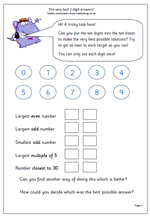I really like this challenge, partly because there is no, one right way to answer it and partly because it really makes children think.

There are 10 digits, from zero to nine to be placed in the 10 boxes in such a way that the targets can be matched as closely as possible. The catch is that each number can only be used once!

Now the obvious way to start is to make 98 the largest even number, but immediately that means that you can not have 97 as the largest odd number. By the time you reach the last target, number closest to 30, you have only two digits left and only two choices!

But what counts as the best possible answers? This is as big a challenge, if not bigger. I have had a class try to make a set of rules to try to be as fair as possible, but it involved a great deal of addition and subtraction. One group made a set of rules that went like this:

1. Find the difference between 98 and the answer given.

2. Find the difference between 99 and the answer given.